# Variational Autoencoder with Tensorflow 2.8 – VII – KL loss via model.add_loss()

I continue my series on options regarding the treatment of the Kullback-Leibler divergence as a loss [KL loss] in Variational Autoencoder [VAE] setups.

Our objective is to find solutions which avoid potential problems with the eager execution mode of present Tensorflow 2 implementations. Popular recipes of some teaching books on ML may lead to non-working codes in present TF2 environments. We have already looked at two working alternatives.

In the last post we transferred the “mu” and “log_var” tensors from the Encoder to the Decoder and fed some Keras standard loss functions with these tensors. These functions could in turn be inserted into the model.compile() statement. The approach was a bit complex because it involved multi-input-output model definitions for the Encoder and Decoder.

The present article will discuss a third and lighter approach – namely using the Keras add_loss() mechanism on the level of a Keras model, i.e. model.add_loss().

The advantage of this function is that its parameter interface is not reduced to the form of standardized Keras cost function interfaces which I used in my last post. This gives us flexibility. A solution based on model.add_loss() is also easy to understand and realize on the programming level. It is, however, an approach which may under certain conditions reduce performance by roughly a factor between 1.3 and 1.5 – which is significant. I admit that I have not yet understood what the reasons are. But the concrete solution version I present below works well.

# The strategy

The way how to use Keras’ add_loss() functionality is described in the Keras documentation. I quote from this part of TF2’s documentation about the use of add_loss():

This method can also be called directly on a Functional Model during construction. In this case, any loss Tensors passed to this Model must be symbolic and be able to be traced back to the model’s Inputs. These losses become part of the model’s topology and are tracked in get_config.

The documentation also contains a simple example. The strategy is to first define a full VAE model with standard mu and log_var layers in the Encoder part – and afterwards add the KL-loss to this model. This is depicted in the following graphics: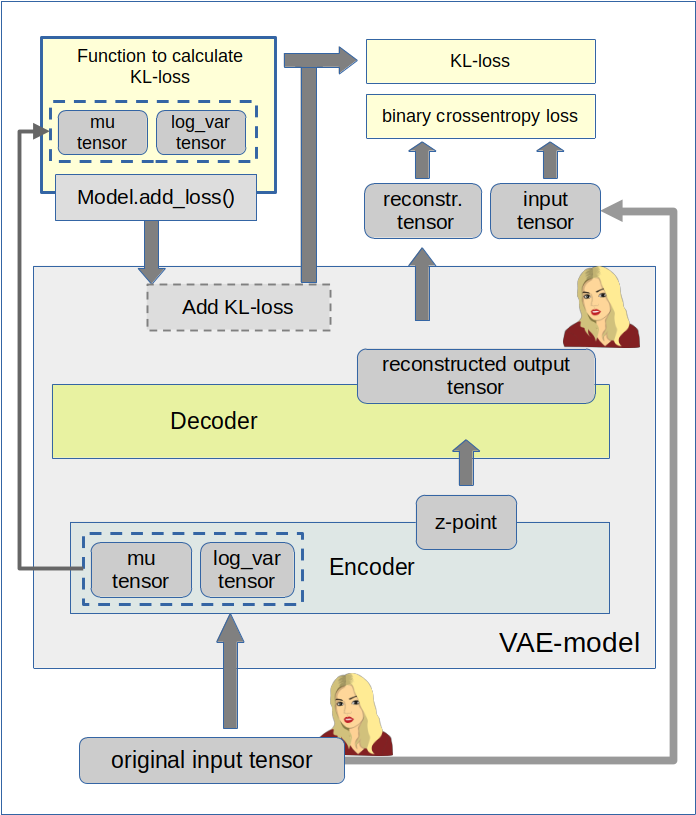We implement this strategy below via the Python class for a VAE setup which we have used already in the last 4 posts of this series. We control the Keras model setup and the layer construction by the parameter “solution_type”, which I have introduced in my last post.

# Cosmetic changes to the Encoder/Decoder parts and the model creation

The class method _build_enc(self, …) can remain as it was defined in the last post. We just have to change the condition for the layer setup as follows:

Change to _build_enc(self, …)

```... # see other posts
...
# The Encoder Model
# ~~~~~~~~~~~~~~~~~~~
# With extra KL layer or with vae.add_loss()
if solution_type == 0 or solution_type == 2:
self.encoder = Model(self._encoder_input, self._encoder_output)

# Transfer solution => Multiple outputs
if solution_type == 1:
self.encoder = Model(inputs=self._encoder_input, outputs=[self._encoder_output, self.mu, self.log_var], name="encoder")
```

Something similar holds for the Decoder part _build_decoder(…):

Change to _build_dec(self, …)

```... # see other posts
...
# The Decoder model
# solution_type == 0/2: Just the decoded input
if self.solution_type == 0 or self.solution_type == 2:
self.decoder = Model(self._decoder_inp_z, self._decoder_output)

# solution_type == 1: The decoded tensor plus the transferred tensors mu and log_var a for the variational distribution
if self.solution_type == 1:
self.decoder = Model([self._decoder_inp_z, self._dec_inp_mu, self._dec_inp_var_log],
[self._decoder_output, self._dec_mu, self._dec_var_log], name="decoder")
```

A similar change is done regarding the model definition in the method _build_VAE(self):

Change to _build_VAE(self)

```        solution_type = self.solution_type

if solution_type == 0 or solution_type == 2:
model_input  = self._encoder_input
model_output = self.decoder(self._encoder_output)
self.model = Model(model_input, model_output, name="vae")

... # see other posts
...
```

# Changes to the method compile_myVAE(self, learning_rate)

More interesting is a function which we add inside the method compile_myVAE(self, learning_rate, …).

Changes to compile_myVAE(self, learning_rate):

```    # Function to compile the full VAE
# ~~~~~~~~~~~~~~~~~~~~~~~~~~~~~~~~~~~~~~~~~~~~~
def compile_myVAE(self, learning_rate):

# Optimizer
# ~~~~~~~~~
# save the learning rate for possible intermediate output to files
self.learning_rate = learning_rate

# Parameter "fact" will be used by the cost functions defined below to scale the KL loss relative to the BCE loss
fact = self.fact

# Function for solution_type == 1
# ~~~~~~~~~~~~~~~~~~~~~~~~~~~~~~~~~
@tf.function
def mu_loss(y_true, y_pred):
loss_mux = fact * tf.reduce_mean(tf.square(y_pred))
return loss_mux

@tf.function
def logvar_loss(y_true, y_pred):
loss_varx = -fact * tf.reduce_mean(1 + y_pred - tf.exp(y_pred))
return loss_varx

# Function for solution_type == 2
# ~~~~~~~~~~~~~~~~~~~~~~~~~~~~~~~~
# We follow an approach described at
# https://www.tensorflow.org/api_docs/python/tf/keras/layers/Layer
# NOTE: We can NOT use @tf.function here
def get_kl_loss(mu, log_var):
kl_loss = -fact * tf.reduce_mean(1 + log_var - tf.square(mu) - tf.exp(log_var))
return kl_loss

# Required operations for solution_type==2 => model.add_loss()
# ~~~~~~~~~~~~~~~~~~~~~~~~~~~~~~~~~~~~~~~~~
res_kl = get_kl_loss(mu=self.mu, log_var=self.log_var)
if self.solution_type == 2:

# Model compilation
# ~~~~~~~~~~~~~~~~~~~~
if self.solution_type == 0 or self.solution_type == 2:
self.model.compile(optimizer=optimizer, loss="binary_crossentropy",
metrics=[tf.keras.metrics.BinaryCrossentropy(name='bce')])

if self.solution_type == 1:
self.model.compile(optimizer=optimizer
, loss={'vae_out_main':'binary_crossentropy', 'vae_out_mu':mu_loss, 'vae_out_var':logvar_loss}
#, metrics={'vae_out_main':tf.keras.metrics.BinaryCrossentropy(name='bce'), 'vae_out_mu':mu_loss, 'vae_out_var': logvar_loss }
)
```

I have supplemented function get_kl_loss(mu, log_var). We explicitly provide the tensors “self.mu” and “self.log_var” via the function’s interface and thus follow one of our basic rules for the Keras add_loss()-functionality (see post IV).
Note that this is a MUST to get a working solution for eager execution mode!

Interestingly, the flexibility of model.add_loss() has a price, too. We can NOT use a @tf.function indicator here – in contrast to the standard cost functions which we used in the last post.

Note also that I have added some metrics to get detailed information about the size of the crossentropy-loss and the KL-loss during training!

# Cosmetic change to the method for training

Eventually we must include solution_type==2 in method train_myVAE(self, x_train, batch_size, …)

Changes to train_myVAE(self, x_train, batch_size,…)

```... # see other posts
...
if self.solution_type == 0 or self.solution_type == 2:
self.model.fit(
x_train
, x_train
, batch_size = batch_size
, shuffle = True
, epochs = epochs
, initial_epoch = initial_epoch
)

if self.solution_type == 1:
self.model.fit(
x_train
#               Working
#                , [x_train, t_mu, t_logvar] # we provide some dummy tensors here
# by dict:
, {'vae_out_main': x_train, 'vae_out_mu': t_mu, 'vae_out_var':t_logvar}
, batch_size = batch_size
, shuffle = True
, epochs = epochs
, initial_epoch = initial_epoch
#, verbose=1
, callbacks=[MyPrinterCallback()]
)

```

# Some results

We can use a slightly adapted version of the Jupyter notebook cells discussed in post V

Cell 6:

```from my_AE_code.models.MyVAE_2 import MyVariationalAutoencoder

z_dim         = 12
solution_type = 2
fact          = 6.5e-4

vae = MyVariationalAutoencoder(
input_dim = (28,28,1)
, encoder_conv_filters = [32,64,128]
, encoder_conv_kernel_size = [3,3,3]
, encoder_conv_strides = [1,2,2]
, decoder_conv_t_filters = [64,32,1]
, decoder_conv_t_kernel_size = [3,3,3]
, decoder_conv_t_strides = [2,2,1]
, z_dim = z_dim
, solution_type = solution_type
, act   = 0
, fact  = fact
)

```

Cell 11:

```BATCH_SIZE = 256
EPOCHS = 37
PRINT_EVERY_N_BATCHES = 100
INITIAL_EPOCH = 0

if solution_type == 2:
vae.train_myVAE(
x_train[0:60000]
, batch_size = BATCH_SIZE
, epochs = EPOCHS
, initial_epoch = INITIAL_EPOCH
)

```

Note that I have changed the BATCH_SIZE to 256 this time; the performance got a bit better then on my old Nvidia 960 GTX:

```Epoch 3/37
235/235 [==============================] - 10s 44ms/step - loss: 0.1135 - bce: 0.1091 - kl: 0.0044
Epoch 4/37
235/235 [==============================] - 10s 44ms/step - loss: 0.1114 - bce: 0.1070 - kl: 0.0044
Epoch 5/37
235/235 [==============================] - 10s 44ms/step - loss: 0.1098 - bce: 0.1055 - kl: 0.0044
Epoch 6/37
235/235 [==============================] - 10s 43ms/step - loss: 0.1085 - bce: 0.1041 - kl: 0.0044
```

This is comparable to data we got for our previous solution approaches. But see an additional section on performance below.

# Some results

As in the last posts I show some results for the MNIST data without many comments. The first plot proves the reconstruction abilities of the VAE for a dimension z-dim=12 of the latent space.

MNIST with z-dim=12 and fact=6.5e-4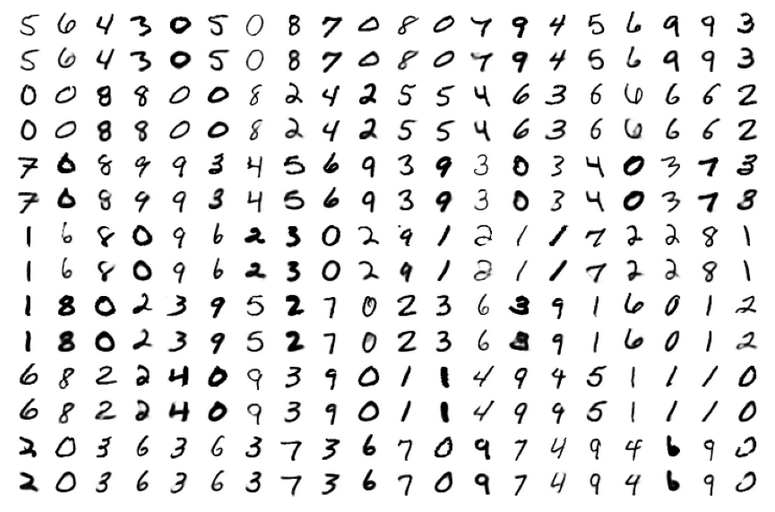For z_dim=2 we get a reasonable data point distribution in the latent space due to the KL loss, but the reconstruction ability suffers, of course:

MNIST with z-dim=2 and fact=6.5e-4 – train data distribution in the z-space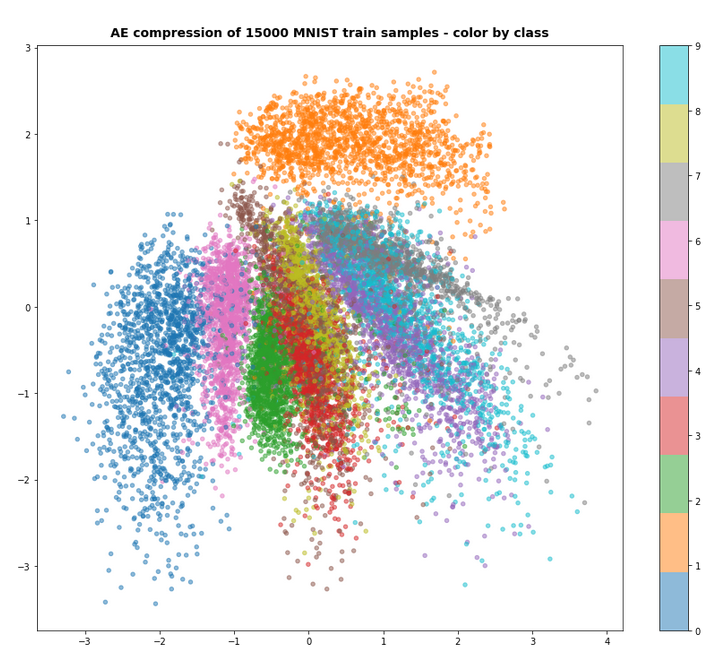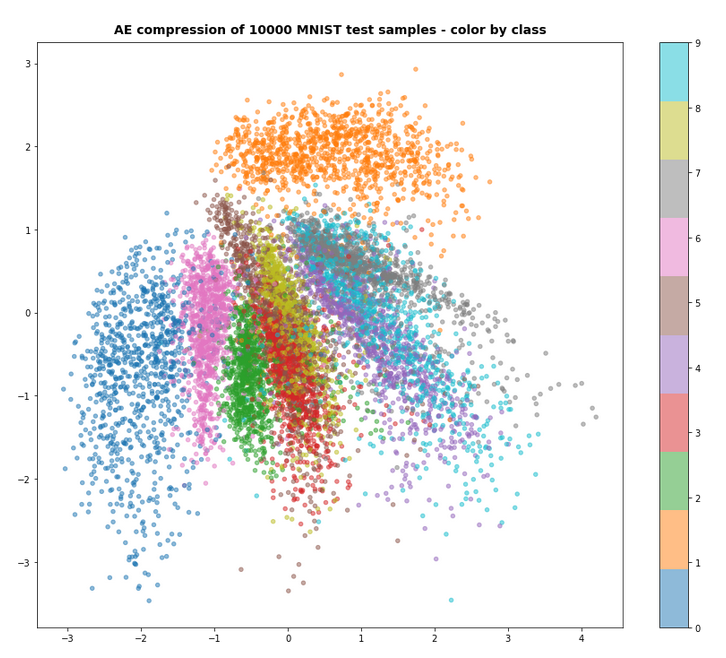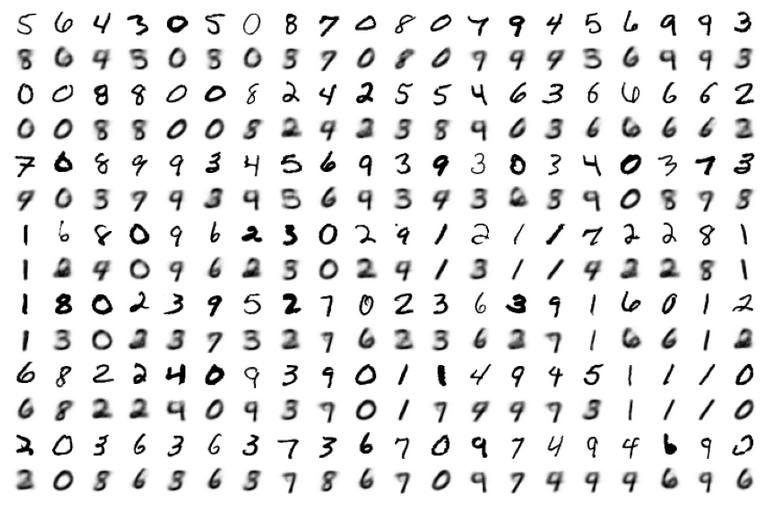For a dimension of z_dim=2 of the latent space and MNIST data we get the following reconstruction chart for data points in a region around the latent space’s origin

# A strange performance problem when no class is used

I also tested a version of the approach with model.add_loss() without encapsulating everything in a class. But with the same definition of the Encoder, the Decoder, the model, etc. But all variables as e.g. mu, log_var were directly kept as data of and in the Jupyter notebook. Then a call

```n_epochs      = 3
batch_size    = 128
initial_epoch = 0
vae.fit( x_train[0:60000],
x_train[0:60000],   # entscheidend !
batch_size=batch_size,
shuffle=True,
epochs = n_epochs,
initial_epoch = initial_epoch
)
```

reduced the performance by a factor of 1.5. I have experimented quite a while. But I have no clue at the moment why this happens and how the effect can be avoided. I assume some strange data handling or data transfer between the Jupyter notebook and the graphics card. I can provide details if some developer is interested.

But as one should encapsulate functionality in classes anyway I have not put efforts in a detail analysis.

# Conclusion

In this article we have studied an approach to handle the Kullback-Leibler loss via the model.add_loss() functionality of Keras. We supplemented our growing class for a VAE with respective methods. All in all the approach is almost more convenient as the solution based on a special layer and layer.add_loss(); see post V.

However, there seems to exist some strange performance problem when you avoid a reasonable encapsulation in a class and do the modell setup directly in Jupyter cells and for Jupyter variables.

In the next post
Variational Autoencoder with Tensorflow 2.8 – VIII – TF 2 GradientTape(), KL loss and metrics
I shall have a look at the solution approach recommended by F. Chollet.

We must provide tensors explicitly to model.add_loss()
https://towardsdatascience.com/shared-models-and-custom-losses-in-tensorflow-2-keras-6776ecb3b3a9

Ceterum censeo: The worst fascist, war criminal and killer living today, who must be isolated, be denazified and imprisoned, is the Putler. Long live a free and democratic Ukraine!

# Variational Autoencoder with Tensorflow 2.8 – IV – simple rules to avoid problems with eager execution

In the last posts of this series

we have seen that it is a bit more difficult to set up a Variational Autoencoder [VAE] with Keras and Tensorflow 2.8 than a pure Autoencoder [AE]. One of the reasons is that we need to include extra layers for a statistical variation of z-points around mean values “mu” with a variance “var” for each sample. In addition a special loss – the Kullback Leibler loss – must be taken into account besides a binary-crossentropy loss to optimize the “mu” and “log_var” values in parallel to a good reconstruction ability of the Decoder.

In the last post we also saw that a too conservative handling of the Kullback-Leibler divergence may lead to problems with the “eager execution mode” of present Tensorflow 2 versions.

In this post I shall first show you how to remedy the specific problem presented in the last post. Sometimes solutions are easy to achieve … :-). But we should also understand the reason for the problem. Some basic considerations will help. Afterward we have a brief look at the performance. At last, we summarize our experiences in some simple rules.

# Eager execution instead of a graph

The next statements are according to my present understanding:
When we designed layered structures of ANNs and related operations with TF 1.x and Keras, Tensorflow built a graph as an intermediate product. The graph contained all mathematical operations in a symbolic way – including the calculation of partial derivatives and gradients. The analysis of the graph by TF afterward lead to a defined sequence of real numerical operations. It is clear that the full knowledge of the graph offers the chance for an optimization of the intended operations, e.g. for ANN-training and error back propagation based on gradient components (=partial derivatives with respect to trainable variables of an ANN, mostly weights). Potential disadvantages of graphs are: Their analysis takes time and it has to be completed before any numerical operations can be started in the background. This in turn means that we cannot test code directly within a sequence of Python statements.

In an eager execution environments planned operations instead are evaluated immediately as the related tensors occur and in case of neural networks as their relation to (weight) variables of interest are properly defined. This includes the calculation of partial derivatives (see my post on error backward calculation for MLPs) with respect to these weights. A requirement is that the operations (= mathematical functions) on specific tensors (represented by matrices) must be well defined. Such operations can be defined by a TF2 math operations directly applied to user defined tensors in a Python statement. But they can also be encapsulated in user or Keras defined functions and combined in complicated ways – provided that it is clear how the chain rule must be applied. As the relation between the trainable variables of neighboring Keras layers in a neural network is well defined also the gradient contributions of two neighbor layers to any loss function is properly defined – and can be calculated already during the forward pass through a neural network. At least in principle we can get resulting tensor values directly or asap during forward propagation wherever possible.

As there are no graphs in eager execution, automatic differentiation based on a graph analysis is not possible without some help. Something has to track operations and functions applied to tensors and record resulting gradient components (i.e. partial derivative values) during a forward pass through a complicated network such that the derivatives can be used during error back-propagation. The tool for this is Gradient.Tape().

A general interface to TF 2.0 like Keras has to incorporate and use Gradient.Tape() internally. While trainable variables like those of a Keras layer can automatically be watched by Gradient.Tape(), specific user defined operations have to be explicitly registered with Gradient.Tape() if you cannot use some Keras model or Keras layer options. However, when you use Keras to define your models gradient related calculations are done directly already during the forward pass through a network. Whilst moving forward through a defined network’s layers gradient contributions (partial derivatives) are evaluated obeying the chain rule across variables of previous layers, of course. The resulting gradient contributions can later be used and properly combined for error backward calculation.

# A remedy to the problem with the failed approach for the KL loss

Just as a reminder: In the last post I introduced a special layer to take care of the KL loss according to a recipe of F. Chollet in his book on Deep Learning of 2017 (see the precise reference at the end of my last post):

Customized Keras layer class:

```class CustVariationalLayer (Layer):

def vae_loss(self, x_inp_img, z_reco_img):
# The references to the layers are resolved outside the function
x = B.flatten(x_inp_img)   # B: tensorflow.keras.backend
z = B.flatten(z_reco_img)

# reconstruction loss per sample
# Note: that this is averaged over all features (e.g.. 784 for MNIST)
reco_loss = tf.keras.metrics.binary_crossentropy(x, z)

# KL loss per sample - we reduce it by a factor of 1.e-3
# to make it comparable to the reco_loss
kln_loss  = -0.5e-4 * B.mean(1 + log_var - B.square(mu) - B.exp(log_var), axis=1)
# mean per batch (axis = 0 is automatically assumed)
return B.mean(reco_loss + kln_loss), B.mean(reco_loss), B.mean(kln_loss)

def call(self, inputs):
inp_img = inputs
out_img = inputs
total_loss, reco_loss, kln_loss = self.vae_loss(inp_img, out_img)
# We add the loss from the layer

return out_img  # not really used in this approach
```

This layer was added on top of the sequence of Encoder and Decoder: Encoder => Decoder => KL_layer.

```enc_output = encoder(encoder_input)
decoder_output = decoder(enc_output)
KL_layer = CustomVariationalLayer()([mu, log_var, encoder_input, decoder_output])
vae = Model(encoder_input, KL_layer, name="vae")
```

# Making it work …

Can we remedy the approach above by some simple means? Yes, we can. I first list the solution’s code, then discuss it:

```# SOLUTION I: Custom Layer for total and KL loss
# ~~~~~~~~~~~~~~~~~~~~~~~~~~~~~~~~~~~~~~~~~~~~~~~
class CustomVariationalLayer (Layer):
def vae_loss(self, mu, log_var, inp_img, out_img):
bce = tf.keras.losses.BinaryCrossentropy()
reco_loss = bce(inp_img, out_img)
kln_loss  = -0.5e-4 * B.mean(1 + log_var - B.square(mu) - B.exp(log_var), axis=1) # mean per sample
return B.mean(reco_loss + kln_loss), B.mean(reco_loss), B.mean(kln_loss) # means per batch

def call(self, inputs):
mu = inputs
log_var = inputs; inp_img = inputs; out_img = inputs
total_loss, reco_loss, kln_loss = self.vae_loss(mu, log_var, inp_img, out_img)
return inputs  # Not used
```

What is the main difference? Answer: We explicitly provided the tensors as input variables of the function vae_loss()!
Why does it help?

Well, TF2 has to prepare and calculate partial derivatives according to the chain rule of differential calculus. What would you yourself want to know on a mathematical level? You would write down any complicated function with further internal operation as a function of well defined arguments! So: We must tell TF2.x explicitly what the variables, namely tensors, of any defined function or operation are to apply the chain rule properly – whatever we do inside the function. When we had graphs this analysis could be done during the analysis of the graph. However, with eager execution we have to know all rules for the affected tensors when they occur and are operated upon. If we operate on tensors via a function, TF2 needs the functions’s arguments to handle the function and following operations properly according to the chain rule. (The tensors themselves at a layer depend, of course, on matrix operations involving trainable parameters, namely weights with respect to a previous layer, and derivatives of activation functions). By the way: The output of the functions must be defined equally well.

In our original approach the function’s input was not defined. It obviously matters with TF2.x!

As a consequence the summary of our VAE model has become longer than in the last post: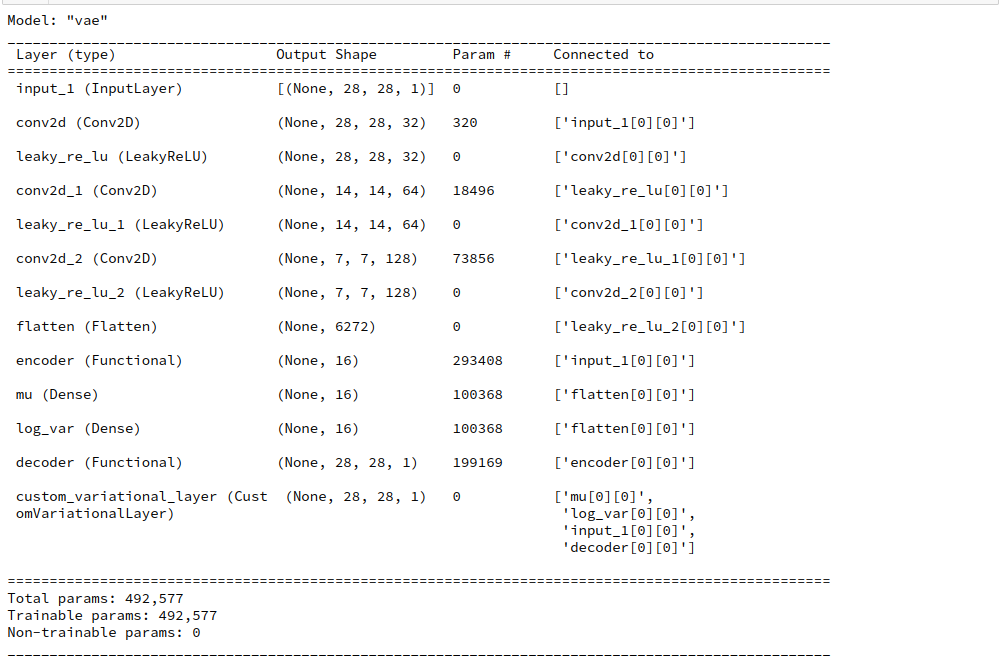# What results do we get for z_dim = 16 and z_dim=2?

For our solution we compile and train like follows:

```vae.compile(optimizer=Adam(), loss=None)
n_epochs = 40
batch_size = 128
vae.fit(x=x_train, y=None, shuffle=True,
epochs = n_epochs, batch_size=batch_size)
```

Note that we do not provide any “y” to fit against. The costs are already fully defined by our special customized layer. If we, however, had used the binary_crossentropy loss in the compile statement we would have had to provide predicted tensors; see below.

On a Nvidia 960 GTX the calculation proceeds for some epochs like: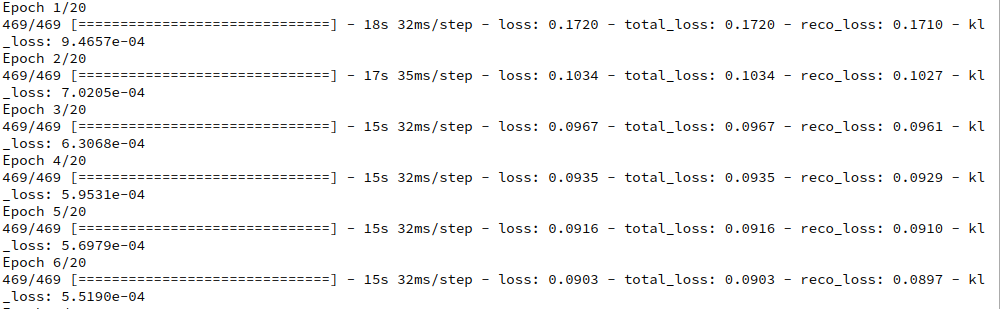After 40 epochs we get with t-SNE well separated clusters for the test-data: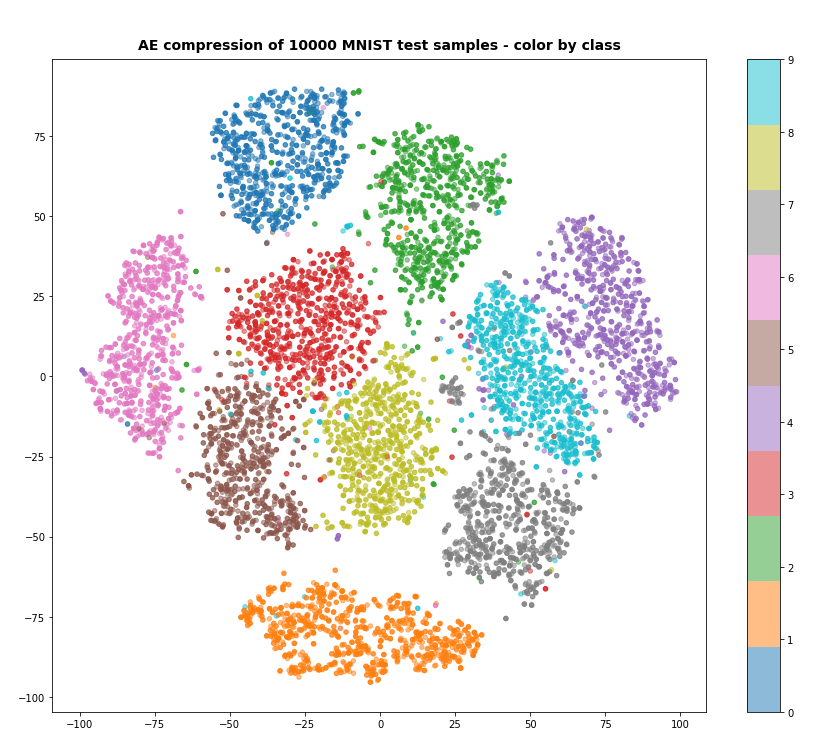More interesting is the result for z_dim = 2, as we expect a more confined usage of the available z-space. And indeed, if we raise the factor in front of the KL loss e.g. to 6.5e-4, we get something like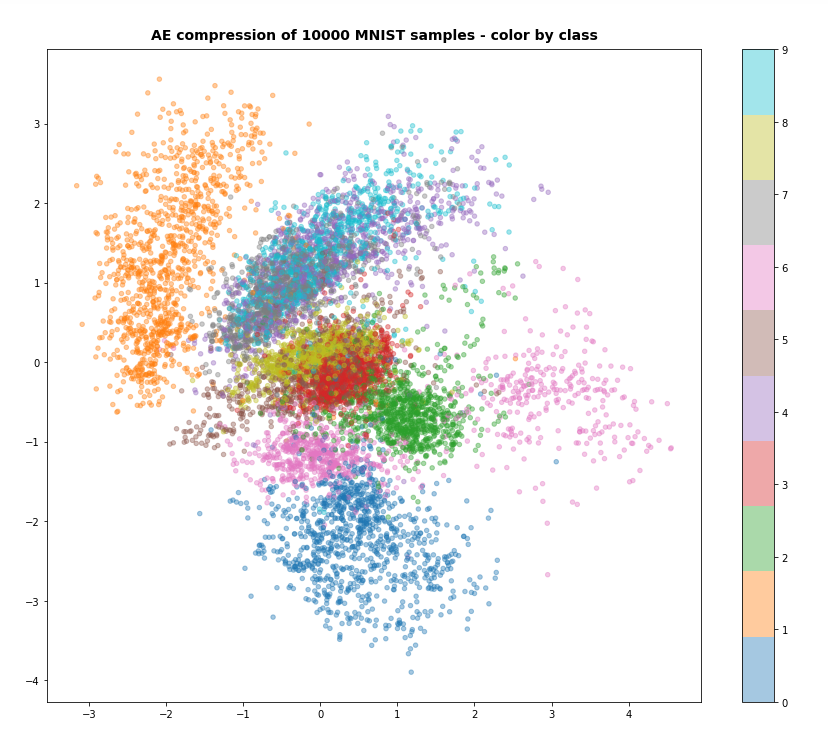With the exception of “6”-digits the samples use the space between -4 < y < 3.5 and -3 < x < 4.5 in z-space. This area is smaller by roughly a factor of 4 (i.e. 2 in each direction) than the space used of a standard Autoencoder (see the 1st post of this series). So, the KL loss shows an effect.

# Performance?

However, our new approach is not as fast as it could be. What can we do to optimize? First we can get rid of the extra function in the layer. We could work directly on the tensors in the call function. A further step would be to focus only on the KL loss. Why not let Keras organize the stuff for binary_crossentropy? But all this would not change our performance much.

The real problem in our case (suggested by the master, F. Chollet, himself in an older book) is an inefficient layer structure: We cannot deal directly with the partial derivatives where the tensors appear – namely in the Encoder. Thereby an otherwise possible sequence of linear algebra operations (matrix operations), which could be optimized for error back propagation, is interrupted in a complicated way at the special layers mu and log_var. So, it appears that a strategy which would encapsulate our KL loss calculation in a specific layer of the Encoder would boost performance. This is indeed the case. I will show the solution in my next post, but give you an idea of the performance gain, already:

Instead of 15 secs as above per epoch we are going to need only 10 to 11 secs.

# What have we learned? Two rules …

I see two basic rules which I personally was not aware of before:

• If you need to perform complex calculations based on layer related tensors to get certain loss contributions and if you want to use the result with pre-defined Keras functions as “layer.add_loss()” and “model.add_loss()” then provide the result tensors explicitly as input variables to the Keras functions. You can use separate personal functions ahead to perform the required tensor operations, but these functions must also have all layer based tensors as explicit input variables and an explicit tensor as output.
• If possible apply your calculations within special layers closely following he layers which provide the tensors your loss contribution depends on. Best before new trainable variables are introduced. Use the special layer’s add_loss() method. Try to verify that your operations fit into a layer related sequence of matrix operations whose values are needed later for error backward propagation, but are calculated already during the forward pass.

The first rule can be symbolized by something like

```# Model definition
...
layer1 = Keras_defined_layer()   #e.g. Dense()
...
layer2 = Keras_defined_layer()   # e.g. Activation()
...
model = Model(....)

# cost calculation
res_tensor_cost_contribution = complex_personal_function( layer1, layer2 )
```

• Try if TF2 math tensor operations are faster than tensorflow.keras.backend operations. I do not think so, but …

# Three strategies to avoid problems with TF 2.8 and VAEs

In the following posts I am going to pursue three ways to handle the KL loss:

1. We add a layer to the Encoder and perform the required KL loss calculation there. We have to take care of a proper output of such a layer not to disrupt the combination of the Encoder with the Decoder. This is in my opinion the most elegant and also the fastest option. It also fits perfectly into the Keras philosophy of defining models via layers. And we can use the Keras compile() and fit() functions seamlessly.
2. We calculate the loss after combining the Encoder and Decoder to a VAE-model – and add the KL loss to our VAE model via its add_loss() method. This is a possible and well defined variant as it separates the loss operations from the VAE’s layer structure. Very similar to what we did above – but probably not the fastest method for VAEs.
3. We use Gradient.Tape() directly to define an individual training step for our Keras based VAE model. This method will prove to be a fast and very flexible method. But in a way it leaves the path of using only Keras layers to define and fit neural network models. Nevertheless: Although it requires a different view on the Keras interface to TF2.x it is certainly the future we should get used to – even if we are no Keras and TF specialists.

# Conclusion

In this post we saw that some old recipes for VAE design with Keras can still be used with some minor modifications. Two rules show us different ways to make Keras based VAE-ANNs work together with TF2.8. In the next post of this series
Variational Autoencoder with Tensorflow 2.8 – V – a customized Encoder layer for the KL loss
we shall build a VAE with an Encoder layer to deal with the Kullback-Leibler loss.